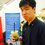# Weekly Math Olympiad Challenge! Problem Set #1

Number Theory

Given positive integers $m$ and $n$, prove that there exists a positive integer $c$ such that the numbers $cm$ and $cn$ have the same occurrences of each non-zero digit in base $10$.

Geometry

Let $ABC$ be a triangle with $\angle A = 90^\circ$. Points $D$ and $E$ lie on sides $AC$ and $AB$, respectively, such that $\angle ABD = \angle DBC$ and $\angle ACE = \angle ECB$. Segments $ED$ and $CE$ meet at $I$. Determine whether or not it is possible for segments $AB, AC, BI, ID, CI, IE$ to all have integer side lengths.

Algebra

Find all functions $f : \mathbb{Z} \rightarrow \mathbb{Z}$ such that for all integers $a, b, c$ that satisfy $a+b+c = 0$, the following equality holds:

$(f(a))^2 + (f(b))^2 + (f(c))^2 = 2f(a)f(b) + 2f(b)f(c) + 2f(a)f(c)$.

Combinatorics

A circle is divided into $432$ congruent arcs by $432$ points. The points are colored with four colors such that some $108$ points are colored each of Red, Blue, Green, and Yellow. Prove that one can choose three points of each color in such a way that the four triangles formed by the chosen points of the same color are congruent.

Extra Credit

Find all positive integers $n$ for which there exists non-negative integers $a_1, a_2, \cdots, a_n$ such that

$\large \frac{1}{2^{a_1}} + \frac{1}{2^{a_2}} + \ldots + \frac{1}{2^{a_n}} = \frac{1}{3^{a_1}} + \frac{2}{3^{a_2}} + \cdots + \frac {n}{3^{a_n}}$

Want a category not included here? Problems are too hard? Too easy? Any feedback would be appreciated!Note by Michael Tong
6 years, 11 months ago

This discussion board is a place to discuss our Daily Challenges and the math and science related to those challenges. Explanations are more than just a solution — they should explain the steps and thinking strategies that you used to obtain the solution. Comments should further the discussion of math and science.

When posting on Brilliant:

• Use the emojis to react to an explanation, whether you're congratulating a job well done , or just really confused .
• Ask specific questions about the challenge or the steps in somebody's explanation. Well-posed questions can add a lot to the discussion, but posting "I don't understand!" doesn't help anyone.
• Try to contribute something new to the discussion, whether it is an extension, generalization or other idea related to the challenge.

MarkdownAppears as
*italics* or _italics_ italics
**bold** or __bold__ bold
- bulleted- list
• bulleted
• list
1. numbered2. list
1. numbered
2. list
Note: you must add a full line of space before and after lists for them to show up correctly
paragraph 1paragraph 2

paragraph 1

paragraph 2

[example link](https://brilliant.org)example link
> This is a quote
This is a quote
    # I indented these lines
# 4 spaces, and now they show
# up as a code block.

print "hello world"
# I indented these lines
# 4 spaces, and now they show
# up as a code block.

print "hello world"
MathAppears as
Remember to wrap math in $$ ... $$ or $ ... $ to ensure proper formatting.
2 \times 3 $2 \times 3$
2^{34} $2^{34}$
a_{i-1} $a_{i-1}$
\frac{2}{3} $\frac{2}{3}$
\sqrt{2} $\sqrt{2}$
\sum_{i=1}^3 $\sum_{i=1}^3$
\sin \theta $\sin \theta$
\boxed{123} $\boxed{123}$

Sort by:

Calculus? Nice problems! Rules for the contest? I couldn't find out how to submit.

- 6 years, 11 months ago

The Algebra and Extra Credit problems are problems 4 and 6 from IMO 2012, aren't they?

- 6 years, 11 months ago

And the NT I believe is a USAMO problem.

- 6 years, 11 months ago

The combinatorics is USAMO 2012 #2 and geometry is USAMO 2010 #4.

And the number theory is 2013 USAMO #5.

@Michael Tong: If you don't want people to have references I'll delete this.

- 6 years, 11 months ago

I don't mind, I trust the community enough to not cheat

This isn't really a competition, it's just for fun and the "challenge." If I wanted to grade it, I would make up problems.

- 6 years, 11 months ago

Well, it's pretty easy to just copy this onto AoPS and find out the sources if wanted. :P

- 6 years, 11 months ago

Wow, Extra Credit Problem is from IMO 2012 P6 and Algebra Problem is from IMO 2012 P4 ......

- 6 years, 11 months ago

Where will we post our answers?

- 6 years, 11 months ago

Okay, thanks for the feedback. I think for answers, people can post their solution (full solution or just a general overview) to comments that I have posted here in the comment section, each corresponding to one question.

- 6 years, 11 months ago

Algebra

- 6 years, 11 months ago

Geometry

- 6 years, 11 months ago

Number Theory

- 6 years, 11 months ago

Combinatorics

- 6 years, 11 months ago

Extra Credit

- 6 years, 11 months ago

Will there be a second set? I had fun solving (or trying to solve) these problems. Nice selection!

- 6 years, 10 months ago

Are there any solutions to these questions???

- 6 years, 10 months ago

You can look the problems up in the Contests page of Art of problem solving

- 6 years, 10 months ago